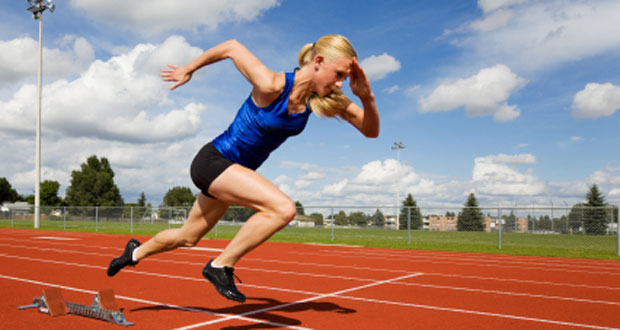# Acceleration & BiomechanicsAcceleration is the rate at which a body changes its velocity and, similarly to velocity, it is a vector quantity which means it has a direction as well as a magnitude. It is measured in m/s/s or metres per second per second (metres per second squared – ms-2).

For example, in the first 30m of a 100m sprint, the sprinter’s velocity will be increasing and will, therefore, have ‘positive acceleration’. Once they have crossed the line, they will begin to slow down during which time they’ll have ‘negative acceleration’ (or deceleration).

## Calculating acceleration

Acceleration can be calculated using the formula below:

Acceleration (ms-2) = Vf – Vi (m/s) / time (s)

(Acceleration = Velocity final – Velocity initial divided by time)

## How does acceleration relate to force?

A body will undergo an acceleration when an external force acts on it. The acceleration will be directly proportional to the force can be calculated by the formula below:

Acceleration (ms-2) = Force (N) / mass (kg)